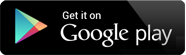## Video Lectures

Chapter 1 : Introduction to Algorithm
Topic : Why to analysis algorithm Running time analysis How to Compare Algorithms Rate of Growth Commonly Used Rates of Growth Types of Analysis Asymptotic Notation Big-O Notation, Omega Notation, Theta, Notation Asymptotic Analysis
Content : Explanation 140 MB ,1:25:31 MIN , THEORY

Chapter 1 : Introduction to Algorithm
Topic : Properties of Notations, Commonly used Logarithms and Summations
Content : Properties of Notations, Commonly used Logarithms and Summations 5 MB ,3:48 MIN , THEORY

Chapter 1 : Introduction to Algorithm
Topic : Master Theorem for Divide and Conquer, Divide and Conquer Master Theorem: Problems & Solutions, Master Theorem for Subtract and Conquer Recurrences
Content : Explanation 108 MB ,1:3:15 MIN , MATHS SOLUTION

Chapter 1 : Introduction to Algorithm
Topic : Method of Guessing and Confirming
Content : Method of Guessing and Confirming 12 MB ,6:31 MIN , THEORY

Chapter 1 : Tree Algorithms
Topic : What is Tree?, Glossary OR Components of Tree, Binary Trees, Types, Properties, Traversals
Content : Explanation 102 MB ,58:16 MIN , THEORY

Chapter 1 : Tree Algorithms
Topic : Generic Trees, Threaded Binary Trees, Expression Trees
Content : Generic Trees, Threaded Binary Trees, Expression Trees 51 MB ,29:43 MIN , THEORY

Chapter 1 : Tree Algorithms
Topic : Binary Search Trees (BSTs)
Content : Binary Search Tree Introduction, Construction, Operations Examples 59 MB ,35:1 MIN , THEORY

Chapter 1 : Tree Algorithms
Topic : Balanced Binary Search Trees, AVL (Adelson-Velskii and Landis) Trees
Content : BST & AVL Tree 49 MB ,28:31 MIN , THEORY

Chapter 2 : Graph Algorithm
Topic : Introduction, Glossary/ Graph Terminologies, Memory Representation
Content : Explanation 73 MB ,43:36 MIN , THEORY

Chapter 2 : Graph Algorithm
Topic : Graph Traversal, Applications
Content : Explanation 74 MB ,43:50 MIN , THEORY

Chapter 2 : Graph Algorithm
Topic : Graph Reachability, Shortest Path, Minimum Spanning Tree
Content : Graph Reachability, Shortest Path, Minimum Spanning Tree 52 MB ,31:48 MIN , THEORY

Chapter 2 : Graph Algorithm
Topic : Topological Sort
Content : Topological Sort 30 MB ,17:34 MIN , THEORY

Chapter 3 : Selection Algorithms
Topic : What are Selection Algorithms?, Selection by Sorting, Partition-based Selection Algorithm, Linear Selection Algorithm - Median of Medians Algorithm, Finding the K Smallest Elements in Sorted Order
Content : What are Selection Algorithms?, Selection by Sorting, Partition-based Selection Algorithm, Linear Selection Algorithm - Median of Medians Algorithm, Finding the K Smallest Elements in Sorted Order 63 MB ,35:46 MIN , THEORY

Chapter 1 : Algorithms Design Techniques
Topic : Introduction, Classification by Implementation Method, Classification by Design Method
Content : Explanation 78 MB ,43:50 MIN , THEORY

Chapter 2 : Greedy Algorithms
Topic : Introduction, Greedy Strategy, Elements of Greedy Algorithms, Advantages and Disadvantages of Greedy Method, Greedy Applications, Understanding Greedy Technique-Huffman Coding Algorithm
Content : Explanation 164 MB ,1:35:47 MIN , THEORY

Chapter 3 : Divide and Conquer Algorithms
Topic : Introduction, Divide and Conquer Visualization, Divide and Conquer Strategy, Understanding Divide and Conquer, Advantages of Divide and Conquer, Disadvantages of Divide and Conquer, Master Theorem Divide and Conquer, Applications
Content : Explanation 64 MB ,38:12 MIN , THEORY

Chapter 4 : Dynamic Programming
Topic : Introduction, What is Dynamic Programming Strategy?, Properties of Dynamic Programming Strategy, Problems which can be solved using Dynamic Programming, Dynamic Programming Approaches, Examples of Dynamic Programming Algorithms, Understanding Dynamic Programming, Longest Common Subsequence
Content : Explanation 106 MB ,1:4:18 MIN , THEORY

## The Shikshak App

The Shikshak App is an honest attempt to provide quality education.